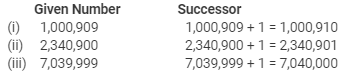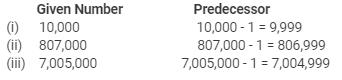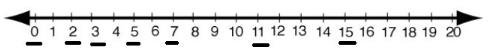Courses

# RD Sharma Solutions (Part - 1) - Whole Number Class 6 Notes | EduRev

## RD Sharma Solutions for Class 6 Mathematics

Created by: Abhishek Kapoor

## Class 6 : RD Sharma Solutions (Part - 1) - Whole Number Class 6 Notes | EduRev

The document RD Sharma Solutions (Part - 1) - Whole Number Class 6 Notes | EduRev is a part of the Class 6 Course RD Sharma Solutions for Class 6 Mathematics.
All you need of Class 6 at this link: Class 6

Page No. 3.4

Ques 1: Write down the smallest natural number.
Ans:
The smallest natural number is 1.

Ques 2: Write down the smallest whole number.
Ans:
The smallest whole number is 0 (zero).

Ques 3: Write down, if possible, the largest natural number.
Ans:
We know that every natural number has a successor. Thus, there is no largest natural number.

Ques 4: Write down, if possible, the largest whole number.
Ans:
We know that every whole number has a successor. Thus, there is no largest whole number.

Ques 5: Are all natural numbers also whole numbers?
Ans:
Yes, all natural numbers are whole numbers.

Ques 6: Are all whole numbers also natural numbers?
Ans:
No, all whole numbers are not natural numbers because 0 is a whole number but not a natural number.

Ques 7: Give successor of each of the whole numbers?
(i) 1000909
(ii) 2340900
(iii) 7039999

Ans:Ques 8: Write down the predecessor of each of the following whole numbers:
(i) 10000
(ii) 807000
(iii) 7005000
Ans:Ques 9: Represent the following numbers on the number line:
2,0,3,5,7,11,15
Ans:Ques 10: How many whole numbers are there between 21 and 61?

Ans: The whole numbers between 21 and 61 are 22, 23, 24, 25, 26, 27, 28, 29, 30, 31, 32, 33, 34, 35, 36, 37, 38, 39, 40, 41, 42, 43, 44, 45, 46, 47, 48, 49, 50, 51, 52, 53, 54, 55, 56, 57, 58, 59 and 60.
Thus, there are 39 whole numbers between 21 and 61.

Ques 11: Fill in the blanks with the appropriate symbol < or >:
(i) 25...205
(ii) 170...107
(iii) 415...514
(iv) 10001...100001
(v) 2300014...2300041
Ans:

We have:
(i) 25 < 205
(ii) 170 > 107
(iii) 415 < 514
(iv) 10001 < 100001
(v) 2300014 < 2300041

Ques 12: Arrange the following numbers is descending order:
925, 786, 1100, 141, 325, 886, 0, 270
Ans:
Numbers in descending order:
1100, 925, 886, 786, 325, 270, 141, 0

Ques 13: Write the largest number of 6 digits and the smallest number of 7 digits. Which one of these two is larger and by how much?
Ans:
Largest six-digit number = 999,999

Smallest seven-digit number = 1,000,000

Thus, the smallest seven-digit number is larger than the largest six-digit number.
Again,
Difference between these two numbers = 1,000,000 - 999,999 = 1
Hence, the smallest seven-digit number is larger than the largest six-digit number by 1.

Ques 14:
Write down three consecutive whole numbers just preceding 8510001.
Ans:
We have:
First number = 8,510,001 - 1 = 8,510,000
Second number = 8,510,000 - 1 = 8,509,999
Third number = 8,509,999 - 1 = 8,509,998
Hence, the three consecutive whole numbers just preceding 8,510,001 are 8,510,000, 8,509,999 and 85,09,998.

Ques 15: Write down the next three consecutive whole numbers starting from 4009998.
Ans:
We have:
First number = 4,009,998 + 1 = 4,009,999
Second number = 4,009,999 + 1 = 4,010,000
Third number = 4,010,000 + 1 = 4,010,001
Hence, the next three consecutive whole numbers starting from 4,009,998 will be 4,009,999, 4,010,000 and 4,010,001.

Ques 16: Give arguments in support of the statement that there does not exist the largest natural number.
Ans:
We know that every natural number has a successor. Therefore, the largest natural number does not exist.

Ques 17: Which of the following statements are true and which are false?
(i) Every whole number has its successor.
(ii) Every whole number has its predecessor.
(iii) 0 is the smallest natural number.
(iv) 1 is the smallest whole number.
(v) 0 is less than every natural number.
(vi) Between any two whole numbers there is a whole number.
(vii) Between any two non-consecutive whole numbers there is a whole number.
(viii) The smallest 5-digit number is the successor of the largest 4 digit number
(ix) Of the given two natural numbers, the one having more digits is greater.
(x) The predecessor of a two digit number cannot be a single digit number.
(xi) If a and b are natural numbers and a < b, than there is a natural number c such that a<b<c.
(xii) If a and b are whole numbers and a<b, then a+1< b+1.
(xiii) The whole number 1 has 0 as predecessor.
(xiv) The natural number 1 has no predecessor.
Ans:
(i) True
The successor of every whole number can be found by adding 1.
(ii) False
Zero (0) is a whole number whose predecessor (-1) is not a whole number.
(iii) False
1 is the smallest natural number.
(iv) False
Zero (0) is the smallest whole number.
(v) True
The smallest natural number is 1, so zero (0) is less than every natural number.
(vi) False

There is no whole number between two consecutive whole numbers.

(vii) True

(viii) True

The smallest five-digit number = 10,000

The largest four-digit number = 9,999

Difference = 10,000 - 9,999 = 1

Because the difference is 1, 10,000 is the successor of 9,999.

(ix) True

(x) False

10 is a two-digit number whose predecessor is 9, which is a one-digit number.

(xi) False

If a and b are consecutive natural numbers, then there cannot be any natural number c in between a and b.

(xii) True

(xiii) True

(xiv) True

The predecessor of natural number 1 is 0, which is not a natural number.

Objective Type Quess

Ques 1: The smallest natural number is
(a) 0
(b) 1
(c) -1
(d) None of these
Ans:
(b) 1

Ques 2: The smallest whole number is
(a) 1
(b) 0
(c) -1
(d) None of these
Ans
:
(b) 0

Ques 3: The predecessor of 1 in natural numbers is
(a) 0
(b) 2

(c) -1

(d) None of these

Ans : (d) None of these
We know that the smallest natural number is 1. Hence, its predecessor does not exist.

Ques 4: The predecessor of 1 in whole numbers is
(a) 0
(b) -1
(c) 2
(d) None of these
Ans
4:

(a) 0
Predecessor of 1 = 1 - 1 = 0

Ques 5: The predecessor of 1 million is
(a) 9999
(b) 99999
(c) 999999
(d) 1000001
Ans:
(c) 9,99,999

We have:

1 million = 10,00,000

Predecessor of 1 million = 10,00,000 - 1  = 9,99,999

Ques 6: The successor of 1 million is
(a) 10001
(b) 100001
(c) 1000001
(d) 10000001
Ans: (c) 10,00,001
We have:

1 million = 10,00,000

Successor of 1 million = 10,00,000 + 1= 10,00,001

Ques 7: The product of the successor and predecessor of 99 is
(a) 9800
(b) 9900
(c) 1099
(d) 9700
Ans:

(a) 9800
We have:
Successor of 99 = 99 + 1 = 100
Predecessor of 99 = 99 − 1 = 98
Their product = 100 × 98 = 9800

Ques 8: The product of a whole number (other than zero) and its successor is
(a)  an even number
(b) an odd number
(c) divisible by 4
(d) divisible by 3
Ans:
(a) an even number
Example:
Whole number = 1
Successor of 1 = 1 + 1 = 2
Their product = 1 × 2 = 2
Thus, 2 is an even number.

103 docs

,

,

,

,

,

,

,

,

,

,

,

,

,

,

,

,

,

,

,

,

,

;